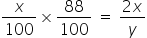# CAT 2021 Slot 1

## CAT 2021 Exam Question Papers Slot 1 | CAT Quants​

### CAT Quantitative Aptitude | CAT 2021 Question Papers​

CAT Question Papers: Solving CAT Question Papers from prior years will give CAT 2022 applicants invaluable insight into the examination format, question pattern, and level of difficulty. The CAT 2021 exams were held in three slots.

It is important for CAT preparation to examine old CAT Exam, Question Paper. In order to overcome the difficulties, they encounter when preparing for the CAT, aspirants must use a variety of strategies.
• By solving CAT Exam previous year question papers, aspirants learn about their own strengths and weaknesses. This allows them to plan their preparation accordingly.
• The secret to passing any exam is practice. Hence, by studying CAT exam papers over the last 5 to 10 years, you can attempt 500+ questions of all types and levels of difficulty.
• They can identify important topics by asking questions that have been asked repeatedly over time.
• CAT aspirants can benefit from numerous study materials and engaging video lessons available at Pathfinder For Me, including Free Download CAT Exam E-Books. Aspirants for the CAT test will get closer to passing the CAT 2022 exam with the help of these CAT question papers with solution PDF.

The best resource for CAT preparation is CAT question papers. Solving previous year’s CAT question papers helps candidates understand the CAT Exam Pattern, types of questions, question weightage, important topics, etc.

All CAT 2021 Quantitative aptitude questions have detailed answer keys and solutions provided by our experts. Watch the videos below to learn the fastest ways to answer the questions:

5).

Identical chocolate pieces are sold in boxes of two sizes, small and large. The large box is sold for twice the price of the small box. If the selling price per gram of chocolate in the large box is 12% less than that in the small box, then the percentage by which the weight of chocolate in the large box exceeds that in the small box is nearest to

1. 127

2. 135

3. 124

4. 144

### Explanation

Let the small box and the large box are sold at x and 2x rupees respectively. Also, let weight of chocolate in small box and large box respectively is 100 gram and y gram respectively. Then

price per gram in small box = x/100 and

price per gram in large box = 2x/y

As per the question,

== > y = 20000/88 = 2500/11 = 227

which is about 127 % more than weight of small box.

Ans (1)

Alternate Method:

Assume Small box = has 100 g of chocolate sold at Rs 1 per gram

Cost of small box = Rs 100

Therefore cost of the large box = Rs 200

Cost price of the chocolate in the large box = 0.88

Quantity = 200/0.88 = 227.27

Thus the large box  has quantity that is (227.27-100)/100 x 100 = 127 % more than the small box